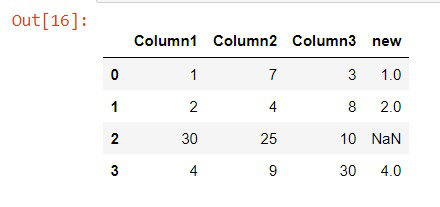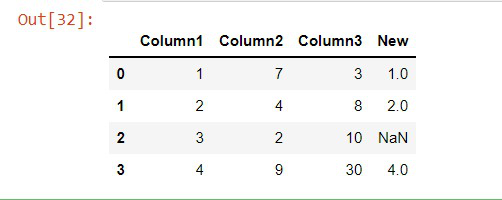Open in App
Not now

# How to Compare Two Columns in Pandas?

• Difficulty Level : Easy
• Last Updated : 20 Dec, 2021

In this article, we learn how to compare the columns in the pandas’ dataframe. Pandas is a very useful library in python, it is mainly used for data analysis, visualization, data cleaning, and many.

Comparing the columns is very needful, when we want to compare the values between them or if we want to know the similarity between them. For example,  if we take two columns, and we want to find which column is greater than or lesser than the other column or also to find the similarity between them, Comparing the column is the suitable thing that we might need to do.  There are many types of methods in pandas and NumPy to compare the values between them, We will see all the methods and implementation in this article.

### Method 1: Using np.where() methods.

In this method, the condition is passed into this method and if the condition is true, then it will be the value we give( that is ‘X in the syntax) if it is false then, it will be the value we give to them (that is ‘y’ in the syntax).

Syntax: numpy.where(condition[,x, y])

Parameters:

• condition : When True, yield x, otherwise yield y.
• x, y : Values from which to choose.

In the below code, we are importing the necessary libraries that are pandas and NumPy. We created a dictionary, and the values for each column are given. Then it is converted into a pandas dataframe. By using the Where() method in NumPy, we are given the condition to compare the columns. If ‘column1’ is lesser than ‘column2’ and ‘column1’ is lesser than the ‘column3’, We print the values of ‘column1’. If the condition fails, we give the value as ‘NaN’.  These results are stored in the new column in the dataframe.

## Python3

 `# Importing Libraries``import` `pandas as pd``import` `numpy as np` `# data's stored in dictionary``details ``=` `{``    ``'Column1'``: [``1``, ``2``, ``30``, ``4``],``    ``'Column2'``: [``7``, ``4``, ``25``, ``9``],``    ``'Column3'``: [``3``, ``8``, ``10``, ``30``]``}` `# creating a Dataframe object``df ``=` `pd.DataFrame(details)` `# Where method to compare the values``# The values were stored in the new column``df[``'new'``] ``=` `np.where((df[``'Column1'``] <``=` `df[``'Column2'``]) & (``    ``df[``'Column1'``] <``=` `df[``'Column3'``]), df[``'Column1'``], np.nan)` `# printing the dataframe``print``(df)`

Output:np.Where()

### Method 2: Using equals() methods.

This method Test whether two-column contain the same elements. This function allows two Series or DataFrames to be compared against each other to see if they have the same shape and elements. NaNs in the same location are considered equal.

Syntax: DataFrame.equals(other)

Parameters: OtherSeries or DataFrame: The other Series or DataFrame to be compared with the first.

Returns:  bool True if all elements are the same in both objects, False otherwise

In the below code, we are following the same procedure, which is importing libraries and creating a dataframe. In this dataframe, I have added a new column that is equal to the ‘column2’ to show what the method does in this dataframe.

## Python3

 `# importing libraries``import` `pandas as pd` `# Storing data in dictionary``details ``=` `{``    ``'Column1'``: [``1``, ``2``, ``3``, ``4``],``    ``'Column2'``: [``7``, ``4``, ``25``, ``9``],``    ``'Column3'``: [``3``, ``8``, ``10``, ``30``],``    ``'Column4'``: [``7``, ``4``, ``25``, ``9``],``}` `# creating a Dataframe object``df ``=` `pd.DataFrame(details)` `df[``'Column4'``].equals(df[``'Column2'``])  ``# Returns True` `# df['Column1'].equals(df['Column2']) Returns False`

Output:

`True`

### Method 3: Using Apply() methods.

This method allows us to pass the function or condition and get to apply the same function throughout the pandas’ dataframe series. This method saves us time and code.

Syntax: DataFrame.apply(func, axis=0, raw=False, result_type=None, args=(), **kwargs)

In the below code, We are repeating the same process to create a dataframe in pandas. By using apply() method we are creating a temporary anonymous function made in apply()  itself using lambda. It checks whether the ‘column1’ is lesser than ‘column2’ and ‘column1’ is lesser than ‘column3’. If it is True it will give ‘column1’ value. If it is False It will print NaN. These values are stored inside the New column. Hence we compared the columns.

## Python3

 `import` `pandas as pd``details ``=` `{``    ``'Column1'``: [``1``, ``2``, ``3``, ``4``],``    ``'Column2'``: [``7``, ``4``, ``2``, ``9``],``    ``'Column3'``: [``3``, ``8``, ``10``, ``30``],``}` `# creating a Dataframe object``df ``=` `pd.DataFrame(details)` `# apply function``df[``'New'``] ``=` `df.``apply``(``lambda` `x: x[``'Column1'``] ``if` `x[``'Column1'``] <``=``                     ``x[``'Column2'``] ``and` `x[``'Column1'``]``                     ``<``=` `x[``'Column3'``] ``else` `np.nan, axis``=``1``)` `# printing the dataframe``print``(df)`

Output:Apply()

My Personal Notes arrow_drop_up